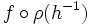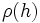# Restricted external wreath product

## Definition

### For an abstract group and a group of permutations

This definition uses the left action convention.

Let$G$ be any abstract group and$H$ be a group along with a homomorphism$\rho:H \to \operatorname{Sym}(S)$ for some set$S$ (in other words, we are given a permutation representation, or group action, of$H$). Then, the restricted external wreath product of$G$ by$H$ (relative to$\rho$) is defined as the external semidirect product of the restricted external direct product$G^S$ (which can be thought of as functions of finite support from$S$ to$G$) by$H$, where an element$h \in H$ sends$f:S \to G$ to the function$f \circ \rho(h^{-1})$.

This wreath product is typically denoted as:$G \wr H$

The group$G$ is termed the base of the wreath product.

The wreath product can also be viewed as follows: The group$G^S$ is the restricted external direct product of$|S|$ copies of$G$, and the action of$h \in H$ is to permute the$|S|$ coordinates by the permutation$\rho(h)$. The significance of restricted direct product is that only finitely many coordinates can be non-identity elements.

There is a related notion of (unrestricted) external wreath product, where we use the unrestricted external direct product. Note that when$S$ is a finite set, these two notions are equivalent.

### For two abstract groups

Let$G,H$ be abstract groups. Then, the restricted external wreath product of$G$ by$H$ is typically understood as the wreath product where the action of$H$ is taken to be the regular group action on itself as a set. In other words, the homomorphism$\rho$ is the natural embedding arising via Cayley's theorem. This wreath product is also termed the restricted regular wreath product.